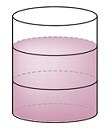# Numerator and denominator of a fraction

👁 107
statistics

Some vocabulary and definitions in fractions

Questions #: 9
Time: 5 minutes
Pass Score: 80.0%
Style
Mode

Missing
POINTS (1)

#### Fraction structure

In a fraction, the number above the (1)is called the (2), and the number below is called the (3).

drag and drop the selected option to the right place or type it instead
fraction bar
denominator
numerator
POINTS (1)

POINTS (1)

POINTS (1)

POINTS (1)

POINTS (1)

POINTS (1)

#### Write fractions that represent the shaded and unshaded portions of the figure belowShaded Fraction = (1)Unshaded Fraction = (2)drag and drop the selected option to the right place or type it instead
$\frac{2}{3}$
$\frac{1}{3}$
POINTS (1)

#### Negative fractions can be written in three ways

Assign the right fraction to each of the following types

1. (1)The negative sign can appear in the numerator
2. (2)The negative sign can appear in the denominator
3. (3)The negative sign can appear in front of the fraction
drag and drop the selected option to the right place or type it instead
$\frac{-1}{2}$
$\frac{1}{-2}$
$-\frac{1}{2}$
POINTS (1)